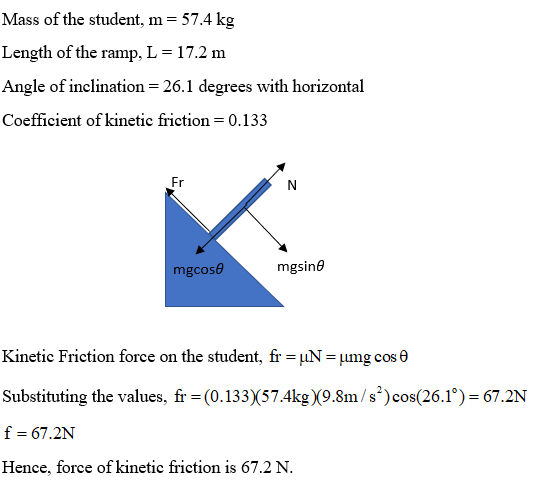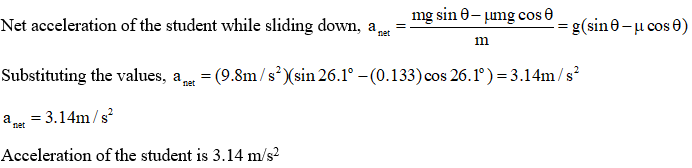# A student of mass 57.4 kg, starting at rest, slides down a slide 17.2 m long, tilted at an angle of 26.1° with respect to the horizontal. If the coefficient of kinetic friction between the student and the slide is 0.133, find the force of kinetic friction, the acceleration, and the speed she is traveling when she reaches the bottom of the slide. (Enter the magnitudes.)

Question
7 views
A student of mass 57.4 kg, starting at rest, slides down a slide 17.2 m long, tilted at an angle of 26.1° with respect to the horizontal. If the coefficient of kinetic friction between the student and the slide is 0.133, find the force of kinetic friction, the acceleration, and the speed she is traveling when she reaches the bottom of the slide. (Enter the magnitudes.)

check_circle

Step 1

Calculating the force of kinetic friction:Step 2

Calculating the acceleration of the student:...

### Want to see the full answer?

See Solution

#### Want to see this answer and more?

Solutions are written by subject experts who are available 24/7. Questions are typically answered within 1 hour.*

See Solution
*Response times may vary by subject and question.
Tagged in

### Physics